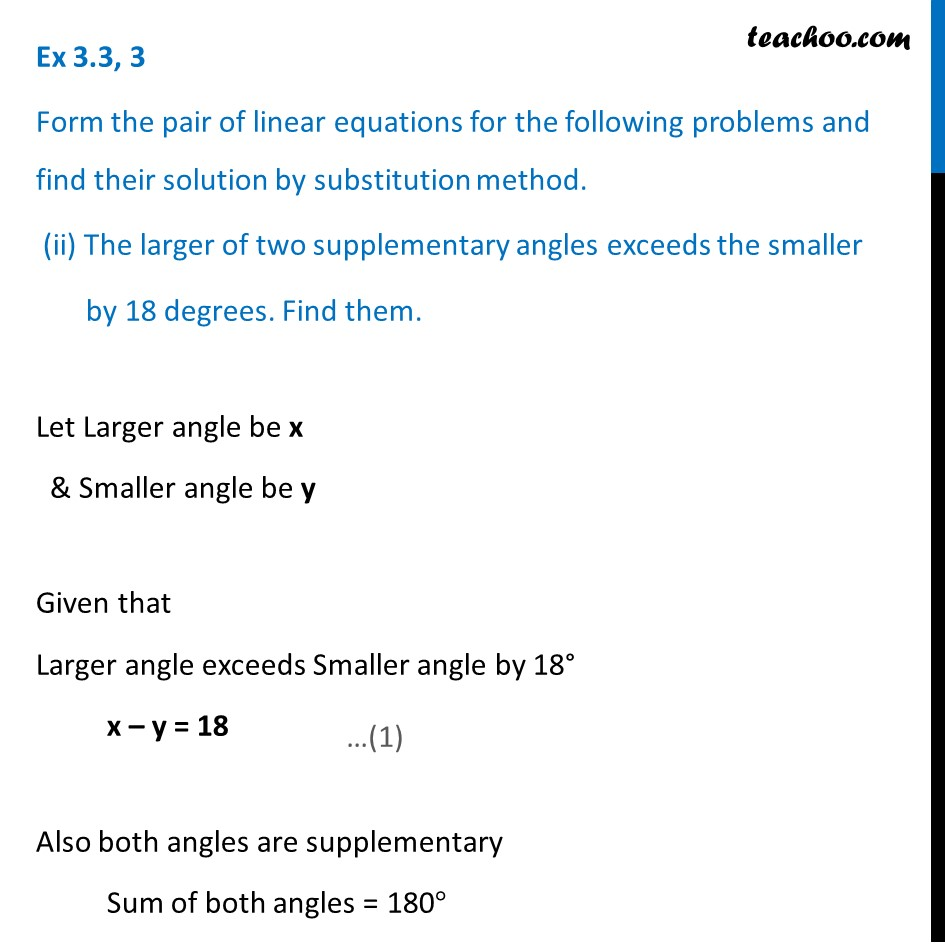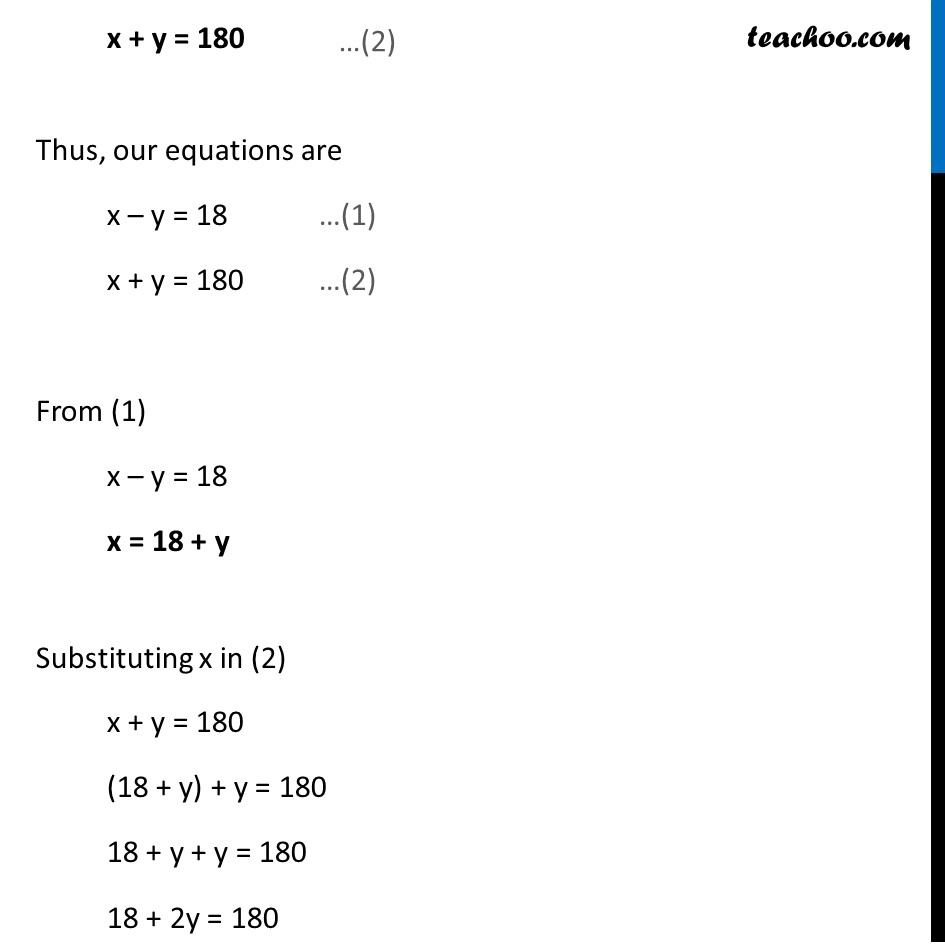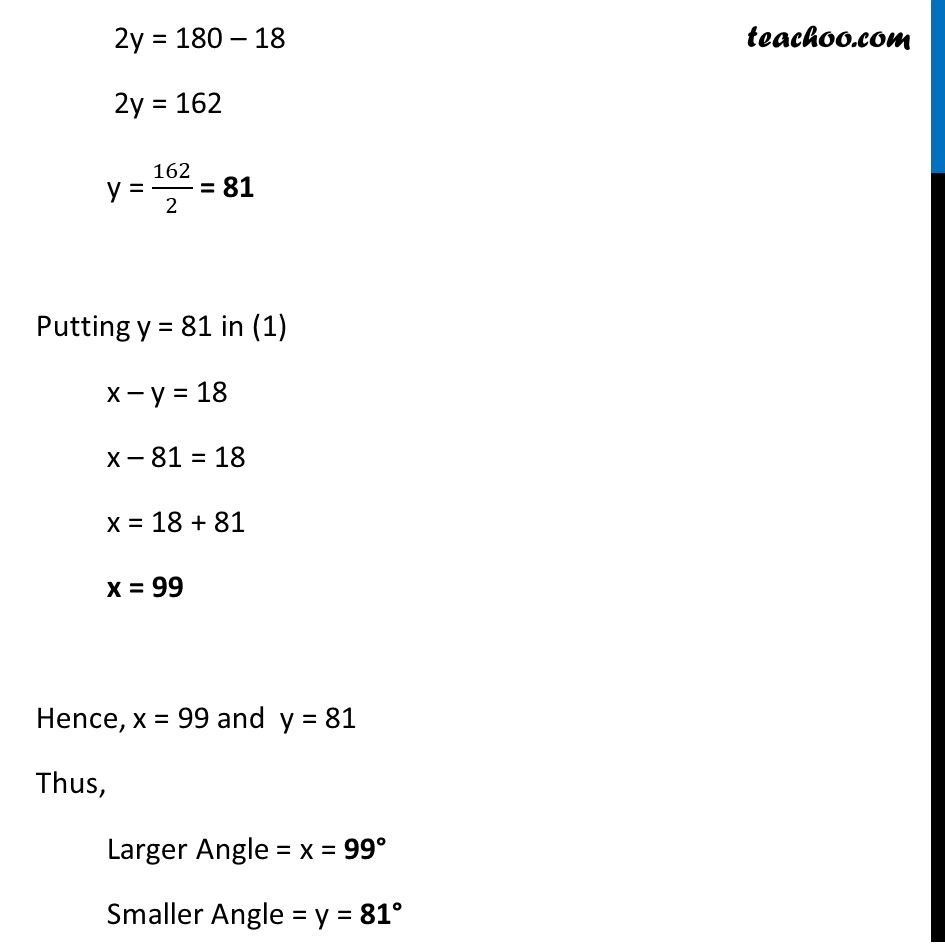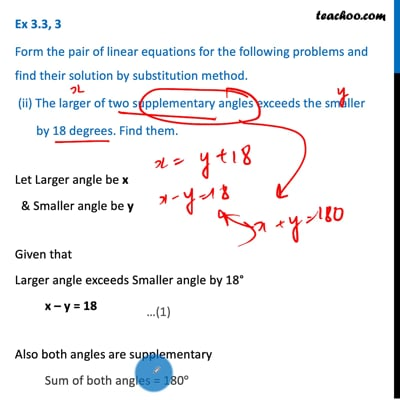Ex 3.3

Chapter 3 Class 10 Pair of Linear Equations in Two Variables (Term 1)
Serial order wiseThis video is only available for Teachoo black users

### Transcript

Ex 3.3, 3 Form the pair of linear equations for the following problems and find their solution by substitution method. (ii) The larger of two supplementary angles exceeds the smaller by 18 degrees. Find them. Let Larger angle be x & Smaller angle be y Given that Larger angle exceeds Smaller angle by 18° x – y = 18 Also both angles are supplementary Sum of both angles = 180° x + y = 180 Thus, our equations are x – y = 18 …(1) x + y = 180 …(2) From (1) x – y = 18 x = 18 + y Substituting x in (2) x + y = 180 (18 + y) + y = 180 18 + y + y = 180 18 + 2y = 180 2y = 180 – 18 2y = 162 y = 162/2 = 81 Putting y = 81 in (1) x – y = 18 x – 81 = 18 x = 18 + 81 x = 99 Hence, x = 99 and y = 81 Thus, Larger Angle = x = 99° Smaller Angle = y = 81°